##### Question

In: Mechanical Engineering

# Water ( ρ= 1000 kg/m3; Cp= 4.2 kJ/kg.K; k= 0.58 W/m.K ) at 1,537 kg/hr and...

Water ( ρ= 1000 kg/m3; Cp= 4.2 kJ/kg.K; k= 0.58 W/m.K ) at 1,537 kg/hr and 26oC enters a 10-mm-diameter smooth tube whose wall temperature is maintained at 79oC. If the water's Nusselt number (Nu) = 375, and the tube length is 7.6, calculate the water outlet temperature,in oC.

## Solutions

##### Expert Solution

The heat transfer is through the tubular surface and therefore the equivalent diameter is equal to the diameter of the tube.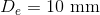The Nusselt number is given by the expression: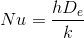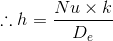Substituting the given values;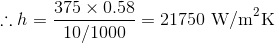Next, we will find the LMTD: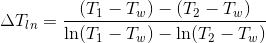Now, we can apply the first law of thermodynamics, i.e., the law of conservation of energy: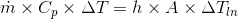Where,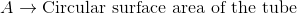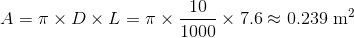Using this: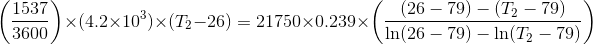This equation cannot be solved analytically, and therefore, needs to be solved numerically.

Using the graphical method, the value of temperature at exit is found as: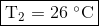## Related Solutions

##### FIreclay brick (ρ=2050 kg/m3, cp=960 J/kg-K, k=1.1 W/m-K) with dimensions 0.06 m x 0.09 m x...
FIreclay brick (ρ=2050 kg/m3, cp=960 J/kg-K, k=1.1 W/m-K) with dimensions 0.06 m x 0.09 m x 0.20 m is removed from a kiln at 1600K and cooled in air at 40C with h = 30 W/m2-K. What is the temperature at the corner of the brick after 50 minutes of cooling? Give your answer in degrees C.
##### Water flowing at a rate of 0.667 kg/s (Cp = 4.192 kJ/kg. K) enters a counter...
Water flowing at a rate of 0.667 kg/s (Cp = 4.192 kJ/kg. K) enters a counter current heat exchanger at 318 K and is heated by an oil stream entering at 393 K at a rate of 2.85 kg/s (Cp=1.89 kJ/kg. K). The overall U=290 W/m2. K and the area A=20 m2. Calculate the heat transfer rate and the exit water temperature.
##### A stainless steel ball (? = 8055 kg/m3 , CP = 480 J/Kg? K ) of...
A stainless steel ball (? = 8055 kg/m3 , CP = 480 J/Kg? K ) of diameter D = 15cm is removed from the oven at a uniform temperature of 350 Degrees C. The ball is then subjected to the flow of air at 1 atm pressure and 30 degrees C with a velocity of 6m/s. The surface temperature of the ball eventually drops to 250 degrees 250 degrees C. Determine the average convection heat transfer coefficient during this cooling...
##### A thick steel slab (ρ= 7800 kg/m3, c= 480 J/kg·K, k= 50 W/m·K) is initially at...
A thick steel slab (ρ= 7800 kg/m3, c= 480 J/kg·K, k= 50 W/m·K) is initially at 300°C and is cooled by water jets impinging on one of its surfaces. The temperature of the water is 25°C, and the jets maintain an extremely large, approximately uniform convection coefficient at the surface. Assuming that the surface is maintained at the temperature of the water throughout the cooling, how long will it take for the temperature to reach 50°C at a distance of...
##### Consider a turbine; working substance is air. cp=1.0 kJ/kgK ,    k=1.4 v1=0.03 ,    v2=0.08 m3/kg ,    ...
Consider a turbine; working substance is air. cp=1.0 kJ/kgK ,    k=1.4 v1=0.03 ,    v2=0.08 m3/kg ,     p1=2.5 bar; state 1 is enterance, state 2 is the exit of the turbine. The turbine is not ideal (isentropic); the air goes through a state change according to pv^n=constant ,      n=1.6 Compute the ideal and actual specific works and turbine efficiency. pvn=constant ,      n=1.6
##### Air (Cp = 1.005 kJ/kg · K) is to be preheated by hot exhaust gases in...
Air (Cp = 1.005 kJ/kg · K) is to be preheated by hot exhaust gases in a cross-flow heat exchanger before it enters the furnace. Air enters the heat exchanger at 95 kPa and 20◦C at a rate of 0.90 kg/s. The combustion gases (Cp = 1.1 kJ/kg · K) enter at a rate of 1.1 kg/s and 150◦C. The product of the overall heat transfer coefficient and the heat transfer surface area is U A = 1200 W/K. Assuming...
##### A gas with cp = 0.950 kJ/kg-K enters an insulated nozzle at 30oC and a velocity...
A gas with cp = 0.950 kJ/kg-K enters an insulated nozzle at 30oC and a velocity of 8 m/s. The gas exits at a velocity of 100 m/s. Assuming constant specific heats and ideal gas behavior, what is the exit temperature of the gas?
##### Air (cp = 1.0 kJ/kgK) enters an air conditioning system at 40C with a mass flow...
Air (cp = 1.0 kJ/kgK) enters an air conditioning system at 40C with a mass flow rate of 1.5 kg/s. The air is cooled by exchanging heat with a stream of R-134a refrigerant that enters the heat exchanger at -8C and 20% quality, and exits with 100% quality. If 25 kW of heat is transferred out of the air, determine: (40 pts) i) Mass flowrate of R-134a (kg/hr) ii) Exit temperature of air (C) iii) Exit pressure of R-134a (kPa)
##### A solution of brine (water containing NaCl) contains 230 kg/m3 at 293 K.
A solution of brine (water containing NaCl) contains 230 kg/m3 at 293 K. The density of the brine at this temperature is 1.148 g/cm3 . Calculate the following, a. composition in mass fractions b. composition in mole fractions c. volume % of water. Assume the density of water at this temperature is 0.998 g/cm3
##### Assume the density of air is ρ = 1.21 kg/m3. A 75-kg skydiver descending head first...
Assume the density of air is ρ = 1.21 kg/m3. A 75-kg skydiver descending head first without parachute will have an area approximately A = 0.18 m2 and a drag coefficient of approximately C=0.70. (a) What would be the predicted terminal velocity of this person if falling from very high altitude (high enough to generate terminal velocity). (b) how many meters above the ground the airplane must fly in order for this person to generate that terminal velocity at the...